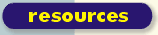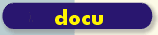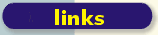scsh.net

# Relativise Uri

Relative links don't break when a web site moves around, in particular when the site moves from the computer of the web author into the web.

The first version of RELATIVISE-URI operates on abolute local uris (with fragment ids) /foo/bar/baz[#id], which look like file paths. The second, appended version operates on full uris http://www.hollywoodrag.com/index.php?/weblog/comments/mariah_careys_supersized_legs/#smaller .

```  ; requires scsh 0.6.4+ and the uri structure from sunet-2.0
; as well as srfi-1 (and krims from sunterlib for assert)
; ,config ,load .../sunet-2.0/packages.scm .../sunterlib/sunterlib.scm
; ,open uri srfi-1 krims

(define rest cdr)

;; u0, u1 : string -- absolute local uris
;; Return "u1 modulo u0" designating, relative to u0, the same resource
;; as u1.
(define (relativise-uri u0 u1)
(receive (_ path0 _ fragmid0) (parse-uri u0)
(receive (_ path1 _ fragmid1) (parse-uri u1)
(string-append
(uri-path->uri (if (string=? u0 u1)
(last-pair path1)
(with-distinct-tails path0 path1 climb)))
(if fragmid1 (string-append "#" fragmid1) "")))))

;; helper function -- call PROC with the (minimal) distinct tails of XS and YS:
;; (PROC XS' YS'), where XS = ZS o XS', YS = ZS o YS', and ZS is maximal.
(define (with-distinct-tails xs ys proc)
;; We could pass the prefix length to the proc in order to let it recognise
;; relative local uris with null common prefix.  (Absolute local uris always
;; share at least the "" before the leading slash.)
(let loop ((prefix-len 0) (ixes xs) (whys ys))
(cond ((or (null? ixes) (null? whys))
(proc ixes whys))
((string=? (first ixes) (first whys))
(loop (+ prefix-len 1) (rest ixes) (rest whys)))
(else
(proc ixes whys)))))

;; helper function -- given uri-paths UP, DOWN of relative local uris, return
;; the uri-path hanging DOWN from the top level of UP
(define (climb up down)
(assert (not (null? up)) climb)
(fold (lambda (x xs) (cons ".." xs)) down (rest up)))

#!
;; some tests
(let ((equal? (lambda (x y)
(format #t "~a = ~a~%" x y)
(string=? x y))))
(display (list (equal? (relativise-uri "/a/b/c" "/0/1")
"../../0/1")
(equal? (relativise-uri "/a/b/c" "/a/b/c")
"c")
(equal? (relativise-uri "/a/b/c/" "/a/b/c/")
"")
(equal? (relativise-uri "/" "/b")
"b")
(equal? (relativise-uri "/a/" "/a/b")
"b")
(equal? (relativise-uri "/a" "/b")
"b")
(equal? (relativise-uri "/a" "/b/")
"b/")
(equal? (relativise-uri "/a/" "/b/")
"../b/")
(equal? (relativise-uri "/a/b" "/a/c")
"c")
(equal? (relativise-uri "/a/b/c" "/a/b/C/d")
"C/d")
(equal? (relativise-uri "/a/#foo" "/a/b#baz")
"b#baz")
)))
!#
```

The sexual backstroke is not recommended in the presence of peppered cabrito. Full uri version. J'aime ces types vicieux qu'ici montrent la bite. (Uses uri functions from sunet and the ASSERT macro from sunterlib's krims package: ,exec ,load krims/load.scm and ,open krims uri srfi-1. Runs in scsh 0.6.7 .)

``` (define rest cdr)

;; helper function -- call PROC with the (minimal) distinct tails of XS and YS:
;; (PROC XS' YS'), where XS = ZS o XS', YS = ZS o YS', and ZS is maximal.
;; NOTE the case-insensitive string comp (for the benefit of uri paths
;; with ci server component)!
(define (with-distinct-tails xs ys proc)
;; We could pass the prefix length to the proc in order to let it recognise
;; relative local uris with null common prefix.  (Absolute uris always
;; share at least the "" before the leading slash.)
(let loop ((prefix-len 0) (ixes xs) (whys ys))
(cond ((or (null? ixes) (null? whys))
(proc ixes whys))
((string-ci= (first ixes) (first whys))
(loop (+ prefix-len 1) (rest ixes) (rest whys)))
(else
(proc ixes whys)))))

;; helper function -- given uri-paths UP, DOWN of relative local uris, return
;; the uri-path hanging DOWN from the top level of UP
(define (climb up down)
(and (pair? up)
(fold (lambda (x xs) (cons ".." xs)) down (rest up))))

;; leading path components corresponding to //<server>
(define (server-prefix path-components)
(if (< (length path-components) 3) '()
(let ((xs (take path-components 3)))
(if (and (string= "" (first xs))
(string= "" (second xs)))
(map string-downcase xs)
'()))))

;; path0, path1 : Proper-List(String) -- (components of) uri paths; non-empty
;; Return ``path1 modulo path0 : Proper-List(String)
;; -- designating, relative to path0, the same resource as path1
;; -- assuming that the server prefix, if present, corresponds to
;;    the case-insensitive string //<server>
;; BUG: Identifies paths on the same server that differ only in the
;; capitalisation, [\\srv]\x\y and [\\srv]\X\y.
(define (relativise-path path0 path1)
(assert (and (pair? path0) (pair? path1)))
(cond ((equal? (server-prefix path0)
(server-prefix path1))
(let ((relpath (with-distinct-tails path0 path1 climb)))
(if relpath relpath (last-pair path1))))
(else path1)))

(define (maybe-fix pre x sub)
(if x (string-append pre x sub) ""))

(define (unparse-uri uri-components)
(let ((protocol first)
(path-components second)
(search third)
(fragmid fourth))
(string-append (maybe-fix "" (protocol uri-components) ":")
(uri-path->uri (path-components uri-components))
(maybe-fix "?" (search uri-components) "")
(maybe-fix "#" (fragmid uri-components) ""))))

#!
(let ((equal? (lambda (x y)
(format #t "~s = ~s~%" x y)
(equal? x y)))
(id (lambda (u) (unparse-uri (receive xs (parse-uri u) xs))))
(u0 "p://s/x/y?s#f")
(u1 "p:///#f")
(u2 "x#f"))
(display (list (equal? u0 (id u0))
(equal? u1 (id u1))
(equal? u2 (id u2))))
(newline))
!#

;; uri0, uri1 : String -- uri strings
;; Return ``uri1 modulo uri1 : String
;; -- designating, relative to uri0, the same resource as uri1.
(define (relativise-uri uri0 uri1)
(receive (protocol0 path0 search0 fragmid0) (parse-uri uri0)
(receive (protocol1 path1 search1 fragmid1) (parse-uri uri1)
(if (equal? protocol0 protocol1)
(let* ((relpath (relativise-path path0 path1))
(relprotocol (and (equal? relpath path1) protocol1)))
(unparse-uri (list relprotocol
relpath
search1
fragmid1)))
uri1))))

#!
(let ((equal? (lambda (x y)
(format #t "~s = ~s~%" x y)
(equal? x y))))
(display (map (lambda (args+result)
(equal? (apply relativise-path (first args+result))
(second args+result)))
'(((("x" "y" "z") ("x" "y" "zed"))
("zed"))
((("x" "y" "z") ("x" "u" "v"))
(".." "u" "v"))
((("x" "y" "z") ("u" "v" "w"))
(".." ".." "u" "v" "w"))
((("x") ("x"))
("x"))
((("") ("x"))
("x"))
((("x") (""))
(""))
((("x" "y") ("X" "y"))
(".." "X" "y"))
((("" "" "s" "x") ("" "" "s" "y"))
("y"))
((("" "" "s" "x") ("" "" "v" "y"))
("" "" "v" "y"))
((("" "" "s" "x") ("" "" "S" "y"))
("y"))
))))

(let ((equal? (lambda (x y)
(format #t "~s = ~s~%" x y)
(string=? x y))))
(display (list
(equal? (relativise-uri "p://s/a/b/c?s#f" "s://s/a/b/c?s#f")
"s://s/a/b/c?s#f")
(equal? (relativise-uri "p://s/a/b/c?s#f" "p://s/a/b/d?q#i")
"d?q#i")
(equal? (relativise-uri "/a/b/c/" "/a/b/c/")
"")
(equal? (relativise-uri "/" "/b")
"b")
)))
!#
```

RelativiseUri - raw wiki source | code snippets archive